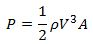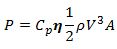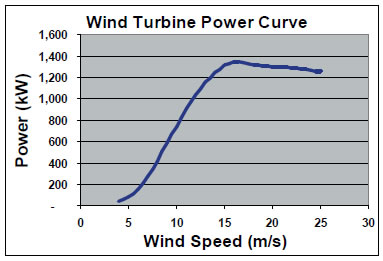### The Power in Wind

The first law of thermodynamics tells us that energy can neither be created nor destroyed, but it can change forms. Anything that is in motion – such as moving air – contains a form of energy we refer to as kinetic energy. Slowing air down reduces its kinetic energy and that energy has to go somewhere. Wind turbines slow wind down and convert some of the energy to mechanical and electrical energy.

Kinetic energy is:Where m stand s for mass (in kg) and V stands for velocity (in m/s). This allows us to calculate the amount of power in moving air. The power of the wind passing perpendicularly through a circular area is:Where:

• P = the power of the wind (in Watts);
• ρ = rho=the density of the air (in kg/m^3), 1.225 kg kg/m^3for dry air at sea level;
• V = the velocity of the wind measured in m/s;
• A=πr^2 = the area swept by the circular rotor, in square meters, and π=pi=3.1415…;
• r= the radius (half the diameter) of the rotor (in meters)

### Betz’s Limit on what we can extract

A wind turbine slows air down, but it cannot remove 100% of the air’s kinetic energy – obviously the air cannot stop completely, or else it would pile up behind the turbine. In 1919, German physicist Albert Betz figured out that the upper limit to the amount of energy you can capture from the wind is 16/27 = 59%. In practice, all real wind turbines extract less than this hypothetical maximum.

### The Power from a Wind Turbine

The power a turbine actually gets from the wind is:You can see that we have added two things to the original equation of the power in the wind:

• Cp = the turbine’s power coefficient;
• η = eta = the turbine’s mechanical & electrical efficiencies;

Cp must be less than the Betz limit. In practice, it varies with wind speed, turbulence and operating characteristics; for example it could be around 44% for a commercial-scale turbine, in winds of 10 m/s. A typical overall efficiency, η, would be in the range of 90%. To estimate the power output of a given commercial turbine, we do not have to use the power equation; rather we use power curves supplied by the manufacturer.### What does the power equation tell us?

Even though we do not use the power equation to calculate power output of a particular turbine, the power equation tells us useful information about significant factors that determine what we can get from a wind turbine:

• V^3: The power available in the wind is proportional to the cube of the wind speed. There is much more energy in high-speed winds than in slow winds. Small changes in changes in wind speed make big changes in power.
• A: Power is proportional to the swept area, and to the square of the diameter. Doubling the diameter quadruples the available power.
• ρ: Air density matters too. The lower density of warmer air, and air at higher altitudes somewhat reduces the power available in the wind.

### References & Resources

• http://www.umass.edu/windenergy/publications/published/communityWindFactSheets/RERL_Fact_Sheet_1_Wind_Technology.pdf